#### You may also like### Golden Thoughts

Rectangle PQRS has X and Y on the edges. Triangles PQY, YRX and XSP have equal areas. Prove X and Y divide the sides of PQRS in the golden ratio.### From All Corners

Straight lines are drawn from each corner of a square to the mid points of the opposite sides. Express the area of the octagon that is formed at the centre as a fraction of the area of the square.### Star Gazing

Find the ratio of the outer shaded area to the inner area for a six pointed star and an eight pointed star.

# The Rescaled Map

##### Age 14 to 16 Challenge Level:

We aim to scale the countries on the map below to represent population size.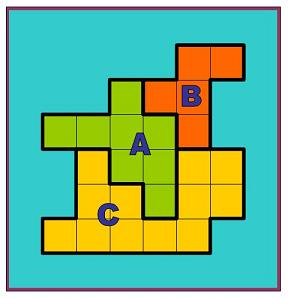We use the hint given and let the ocean keep its original proportion of the map, which is $\frac{39}{64}\times 100=61$ of the map.

 Country Area (million square kilometres) Proportion of total land area Population (millions) Proportion of total population A 8 0.32 40 0.4 B 5 0.2 36 0.36 C 12 0.48 24 0.24

We then find out the factors by which the country sizes need to change:

A needs to cover $\frac{0.4}{0.32}=1.25$ times more area on the map

B needs to cover $\frac{0.36}{0.2}=1.8$ times more area on the map

C needs to cover $\frac{0.24}{0.48}=0.5$ times less area on the map

So we first stretch each country by its scale factor in the vertical direction. For example, the country B would have new dimensions as below: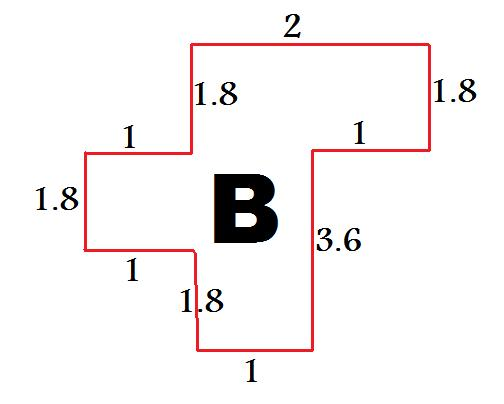And so the overall map becomes (each square has dimensions 0.5 x 0.5)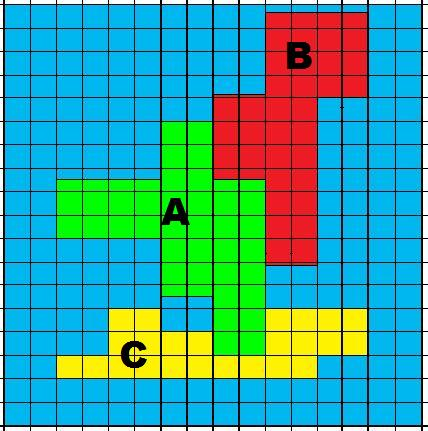We now scale in the horizontal direction, and for example the country C (marked in yellow) would have new dimensions as below: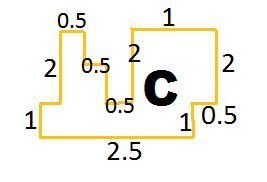And so the overall map becomes (each square again with dimensions 0.5 x 0.5)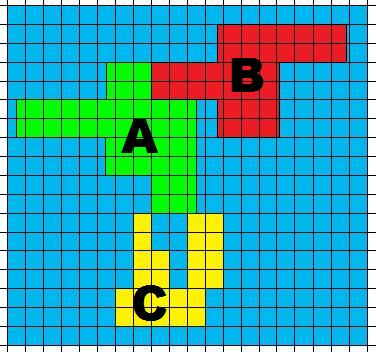Now we combine the scaling factors in both the horizontal and vertical directions. For example, the country A would have dimensions as below: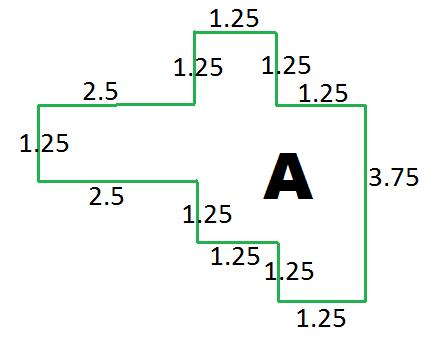Well done to Jack from the King John School, who made a good attempt at resizing the countries in both directions. We leave this part for you to try! Perhaps scale countries B and C next, and then draw them on a map, making sure the ratio of land to ocean is kept constant.